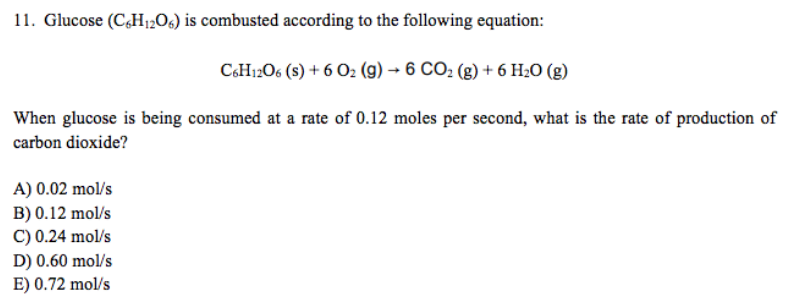# Problem: Glucose (C6H12O6) is combusted according to the following equation: C6H12O6 (s) + 6O2 (g) → 6CO2 (g) + 6H2O (g) When glucose is being consumed at a rate of 0.12 moles per second, what is the rate of production of carbon dioxide? A) 0.02 mol/s B) 0.12 mol/s C) 0.24 mol/s D) 0.60 mol/s E) 0.72 mol/s

🤓 Based on our data, we think this question is relevant for Professor Stepan's class at PSU.

###### FREE Expert Solution###### Problem Details

Glucose (C6H12O6) is combusted according to the following equation:

C6H12O6 (s) + 6O2 (g) → 6CO2 (g) + 6H2O (g)

When glucose is being consumed at a rate of 0.12 moles per second, what is the rate of production of carbon dioxide?

A) 0.02 mol/s

B) 0.12 mol/s

C) 0.24 mol/s

D) 0.60 mol/s

E) 0.72 mol/s